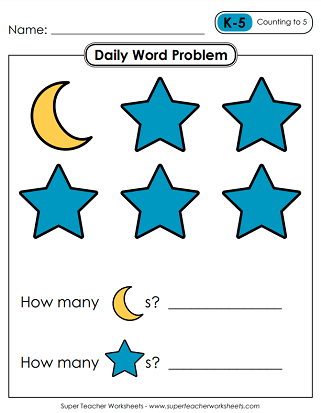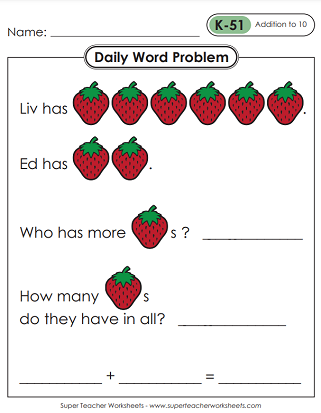# Daily Word Problems - Level K (Kindergarten)

We have a set of Daily Math Word Problem worksheets for Kindergartners. Skills include counting, comparing (more/less), and basic addition/subtraction. Use these worksheets to supplement your math lessons, for morning work, or as part of your classroom meeting routine.This file contains 5 graphical math word problems for Kindergarten students, one for each day of the school week. These basic problems will allow children to practice counting groups of animals and comparing numbers (more/less).
Kindergarten
In the 2nd week, students will count up to 10 and compare groups of musical notes, fish, bananas, and soccer balls. They'll also compare objects using the words more and most.
Kindergarten
There's more counting and comparing in Week #3. Count and compare symbols (hearts, diamonds), pennies, and snap cubes (Unifix cubes). Also determine which single-digit number has the smallest value.
Kindergarten
Add red and blue circular counters on a five-frame. Count and compare marbles, fruit, and weather symbols. Find the total sum of the snap (Unifix) cubes.
Kindergarten
In the 5th week of our daily word problem series, your students will add to find sums within 5, compare groups of objects, and determine the 1-digit number with the largest value.
Kindergarten
Add to calculate the number of counters of a tens chart. Determine which number has the greatest value. Solve word problems with illustrations.
Kindergarten
On these word problems, students will count the number of sides on a polygon, add pencils, and add bundles of sticks by skip counting.
Kindergarten
Solve two very basic subtraction problems with illustrations: (3-1=2) and (4-2=2). Add counters on a ten frame. Identify a trapezoid and count its sides.
Kindergarten
Skip count by tens. Then add and subtract using illustrations and tens charts. Answer questions related to most and fewest objects.
Kindergarten
Find a pair of numbers that make ten, using a tens chart. Count bundles of dowel rods by tens. Count the sides on a hexagon. Add and subtract.
Kindergarten
Determine who has more and who has less. Use illustrations to subtract coins. Identify a rectangle and count the sides. Count to 70 by 10s.
Kindergarten
Compare using the words most and fewest as well as tallest and shortest. Add using pictures of beads and unifix snap cubes. Subtract using pictures of fish.
Kindergarten
With one worksheet for each day of the week, students will practice key kindergarten math skills such as largest and smallest value, addition to 10, and more.
Kindergarten
This set of daily word problems will reinforce key kindergarten math skills--from identifying basic shapes to basic subtraction.
Kindergarten
Use this set of daily word problems to help students practice skills such as comparing (fewest/most), basic shape recognition, counting, and more.
Kindergarten
Kindergarten Resources

We have a huge selection of Kindergarten teaching resources. Topics include basic shapes, early literacy, phonics, counting, hundreds charts, patterns, scissor skills, and much more!

More Daily Word Problems

STW also has Daily Word Problems for students in 1st, 2nd, 3rd, and 4th grades. Take a look!

## Sample Worksheet ImagesMy Account
Site Information